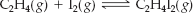×
Get Full Access to Introductory Chemistry - 5 Edition - Chapter 15 - Problem 96p
Get Full Access to Introductory Chemistry - 5 Edition - Chapter 15 - Problem 96p

×

# This reaction is endothermie. If you were a chemist trying to maximize the amount ofISBN: 9780321910295 34

## Solution for problem 96P Chapter 15

Introductory Chemistry | 5th Edition

• Textbook Solutions
• 2901 Step-by-step solutions solved by professors and subject experts
• Get 24/7 help from StudySoup virtual teaching assistantsIntroductory Chemistry | 5th Edition

4 5 1 354 Reviews
19
0
Problem 96P

This reaction is endothermie.If you were a chemist trying to maximize the amount of C2H4I2 produced, which of the following might you try? Assume that the reaction mixture reaches equilibrium.

(a) decreasing the reaction volume

(b) removing I2 from the reaction mixture

(c) raising the reaction temperature

(d) adding C2H4 to the reaction mixture

Step-by-Step Solution:
Step 1 of 3

This reaction is endothermic.(a) decreasing the reaction volume

Decreasing reaction volume will shift to the right because more moles of product (1) vs reagents (2) is favored when there is a decrease in volume.

In other words, the reaction shifts in...

Step 2 of 3

Step 3 of 3

##### ISBN: 9780321910295

This textbook survival guide was created for the textbook: Introductory Chemistry, edition: 5. Since the solution to 96P from 15 chapter was answered, more than 752 students have viewed the full step-by-step answer. The answer to “This reaction is endothermie. If you were a chemist trying to maximize the amount of C2H4I2 produced, which of the following might you try? Assume that the reaction mixture reaches equilibrium.(a) decreasing the reaction volume(b) removing I2 from the reaction mixture(c) raising the reaction temperature(d) adding C2H4 to the reaction mixture” is broken down into a number of easy to follow steps, and 51 words. This full solution covers the following key subjects: reaction, mixture, produced, chemist, decreasing. This expansive textbook survival guide covers 19 chapters, and 2045 solutions. The full step-by-step solution to problem: 96P from chapter: 15 was answered by , our top Chemistry solution expert on 05/06/17, 06:45PM. Introductory Chemistry was written by and is associated to the ISBN: 9780321910295.

Unlock Textbook Solution